Next: Limb Length and Intensity Up: Mouth Localization Previous: Horizontal Symmetry Projection

## Limb Extraction

Kelly proposes the linking of adjacent points in the axial symmetry maps as a means of recovering complete limb structures from symmetry data . This process is simplified when only horizontally aligned symmetry lines are to be considered since limb extraction is reduced to a 3 dimensional linking process. Limb extraction involves grouping points that are adjacent in the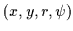space into continuous lines. However, since we are only considering the horizontally projected symmetry lines, we only need to connect points in a (x,y,r) space. Adjacent points in the axial symmetry maps are linked using connected components analysis. Note that adjacent not only refers to spatial adjacency but also adjacency in scale-space (i.e, neighbouring values of r).

Since we are searching specifically for mouths, the connected component analysis can be further constrained. Not only is the symmetry data projected onto the horizontal, it is to be linked horizontally as well. We are searching specifically for connected horizontal limbs and not an arbitrary cloud of adjacent points from the images in Figure. The extraction will produce a set of 3D curves that flow through the symmetry data in Figure. Figuredisplays the limb axes (shown with dashed lines) that should approximate the clouds (or manifolds) of symmetry points (enclosed with continuous lines) in the search space.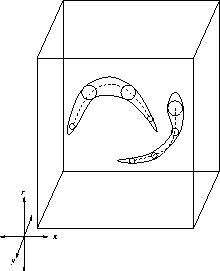Furthermore, these 3D curves are to be as horizontal as possible and should not meander excessively. The mouth is a smooth limb so its axis should be a simple straight line or a slight curve. Furthermore, axial symmetry curves should not undulate excessively in scale-space, r (the third dimension). This prevents the vertical thickness of the mouth limb from varying wildly. Thus, mouth limbs which are maximally straight will be favored. Figuredisplays limb extractions which do not satisfy these criteria and hence are probably not mouths. Such limbs should be attenuated during extraction so that they will not interfere with mouth detection. This attenuation agrees with the Gestalt psychology notion of Pragnanz'' which identifies a correspondence between simplicity in image structures and their perceptual significance .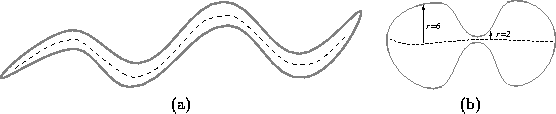Connected component analysis begins at a given point (p,r) in the 3D search space. This 3D search space covers values r=1 to r=6 and the (x,y)region depicted in Figure. This starting point ((p,r) or (x,y,r)) will be called the seed''. One of the requirements we impose is that the seed has a significant horizontal symmetry magnitude (25% of the maximum possible magnitude). From this starting point, we form a limb or 3D curve by propagating along two trajectories: to the left and to the right.

By left propagation, we seek a path towards the left of the current pixel (p,r) which flows through the strongest horizontal symmetry points in our 3D search space. Along the left propagation, we utilize a kernel that covers all the adjacent neighbours of the current point (p,r)=(x,y,r) to the left of the current pixel. This kernel is depicted in Figure. This kernel contains 9 cells of which we must pick one. This new cell becomes the new current cell'' and we repeat the process, tracing out the next step in the path from the 9 possible choices in the kernel. This propagation is continued and the trajectory flows through the 3D space as it repeatedly selects from the 9 possible cells in the kernel.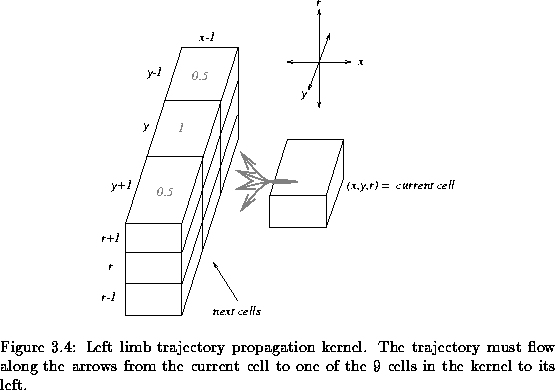The symmetry magnitudes are scaled by the weights depicted in the kernel in Figure. We thus measure a weighted symmetry value from the horizontal projection data for each cell in the kernel. The pixel location to the immediate left is favored most and its symmetry magnitude is consequently scaled by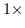. We simultaneously disfavor diagonally positioned pixels by scaling their magnitude by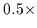. We begin by only considering the spatial neighbourhood at scale r. The peak weighted response from the three cells at r is determined. If it is greater than the threshold (25%) then we move (propagate) to the strongest cell's position and re-compute the kernel from there. If on the other hand, the scale at r has only weak symmetry, we repeat the analysis at r+1 and r-1 and propagate to the strongest of those 6 cells. Thus, we seek a path towards the left of the current pixel which flows through the strongest horizontal symmetry. However, we favor paths that are horizontal (limiting spatial meandering) and paths that are at the same scale (limiting meandering in scale). Equationillustrates the weighted computation of the peak-response of the strongest cell in the kernel. The cell generating the peak-response, peak is the one we move to next. It becomes the current cell'' and we repeat the kernel computation from there. The process stops when a dead end is reached and none of the 9 possible cells contain an output greater than the threshold (25%). In Equation, the horizontal symmetry values are labelled S where S(x,y,r)=Srhorizontal(x,y).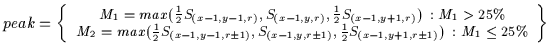(3.3)

A similar propagation is performed to the right of the seed (or starting cell) and the two trajectories are merged into one. This single trajectory in the spatial and scale domain represents the extracted limb as a whole.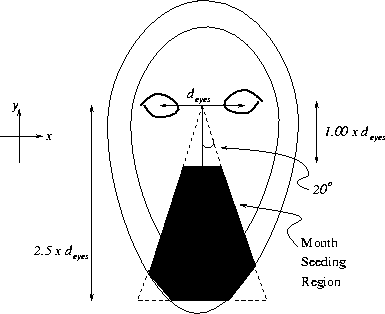Figureshows (in black) the (x,y) region in the image where the mouth limb starting points will be selected. The dimensions of this triangular search region are defined in the figure. Note that the triangle does not extend beyond the face mask so that the mouth search is performed exclusively within the face contour. Any part of the mouth that intersects this triangular search space will trigger limb extraction. Thus, the mouth does not have to be perfectly centered upon the face's mid-line. This can happen if the face has an unusual pose or the eye detection is slightly inaccurate. The triangular search space is superimposed upon the rotated intensity image in Figure. Observe how the mouth is not perfectly aligned with the face's mid-line in Figure. However, it still falls within the generous triangular search region and is thus detectable.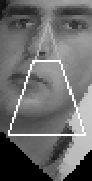Note that this triangular search space is rather redundant. Say a horizontal limb is detected at a seed position (x,y,r) on the image. A very similar limb will probably be detected when we start the limb extraction at (x+1,y,r). Thus, we do not need to start the limb extraction process at every point in the triangle. We merely start the limb extraction once for every value of y in the triangle. For each value of y we select the seeding point (x,y,r) by varying x and r to maximize Srhorizontal(x,y). Then, we begin the limb extraction and extract via propagation the trajectory of the limb towards the left and the right. This is repeated for each value of y in the triangle resulting in a collection of limb axes.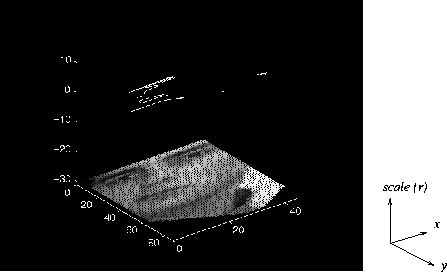The resulting horizontal limb axes are stored as 3D curves (or trajectories) in the spatial-scale domain as shown in Figure. The height in this graph represents the scale or the dimension r of the trajectory. This value of r corresponds directly to the thickness of the limb being extracted. Now, we must test these limbs to determine which of them is the mouth. Some particularly short limbs are present in Figuredespite the Gaussian filtering. These limbs will be discarded later since they are too short.Next: Limb Length and Intensity Up: Mouth Localization Previous: Horizontal Symmetry Projection
Tony Jebara
2000-06-23